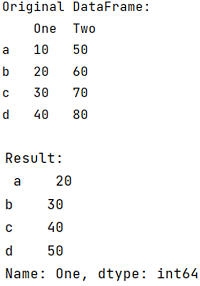# Lambda including if, elif and else

Learn about the Lambda including if, elif and else in Python. By Pranit Sharma Last updated : September 24, 2023

Pandas is a special tool that allows us to perform complex manipulations of data effectively and efficiently. Inside pandas, we mostly deal with a dataset in the form of DataFrame. DataFrames are 2-dimensional data structures in pandas. DataFrames consist of rows, columns, and data.

## Lambda including if, elif and else

To use the lambda function including if, elif, and else, we need to use the lambda() function. Lambda function is like a comprehension function inside which any variable can be defined and that variable is also manipulated in the same line using any particular operator.

Note

To work with pandas, we need to import pandas package first, below is the syntax:

```import pandas as pd
```

Let us understand with the help of an example,

## Python program for 'Lambda including if, elif and else'

```# Importing pandas package
import pandas as pd

# Creating a dictionary
d = {
'One':[10,20,30,40],
'Two':[50,60,70,80]
}

# Creating a DataFrame
df = pd.DataFrame(d,index=['a','b','c','d'])

# Display original DataFrame
print("Original DataFrame:\n",df,"\n")

# Applying lambda function
result = df['One'].apply(lambda x: x*10 if x<2 else (x**2 if x<4 else x+10))

# Display result
print("Result:\n",result)
```

### Output

The output of the above program is: